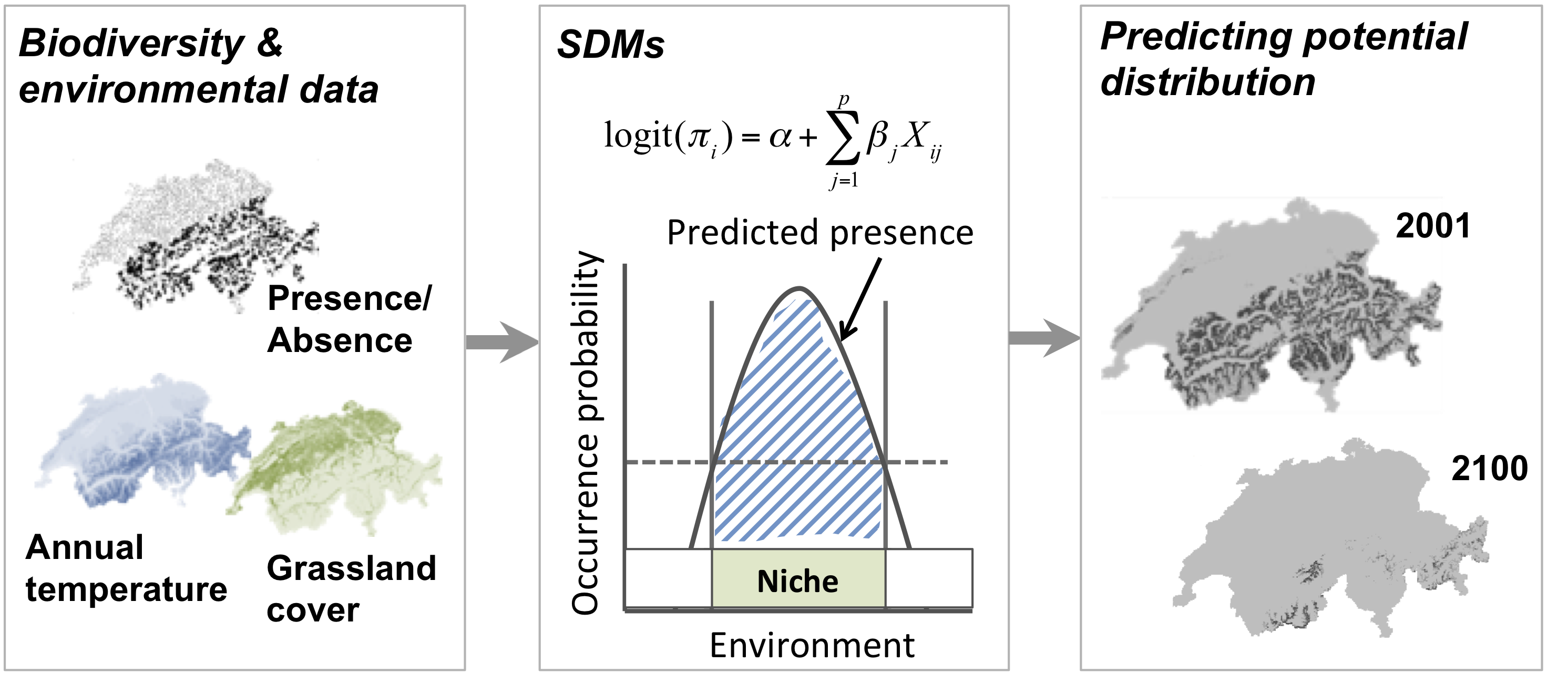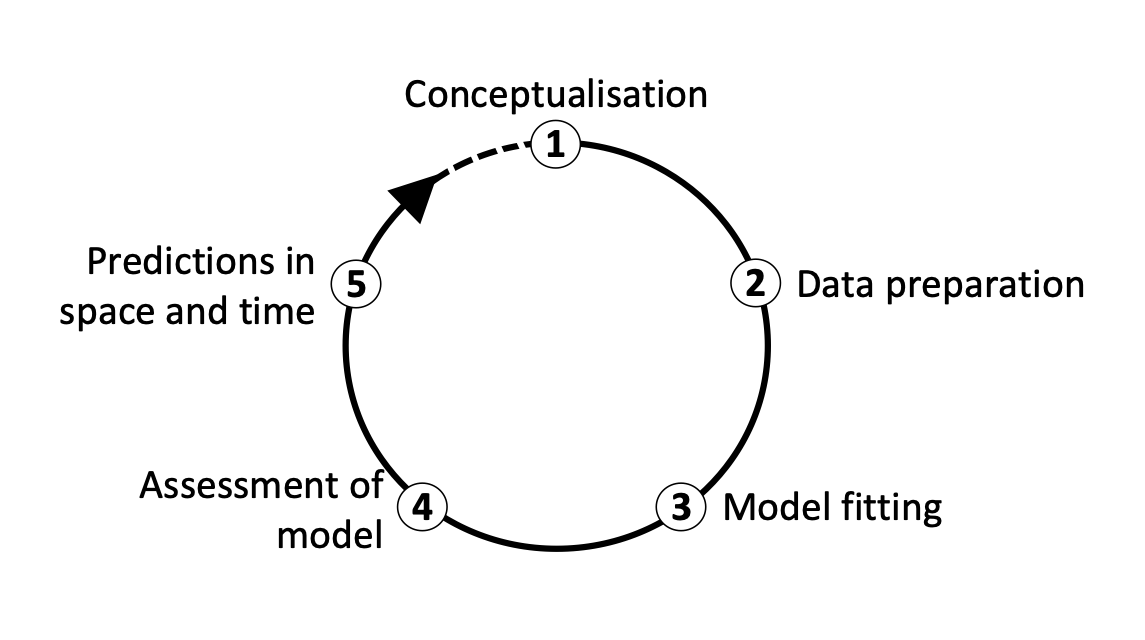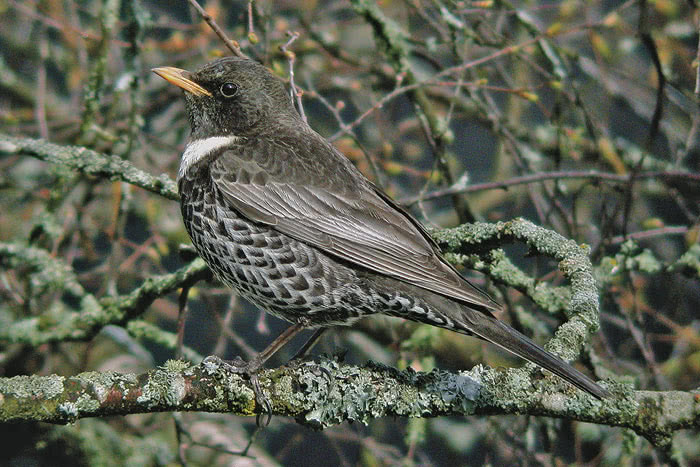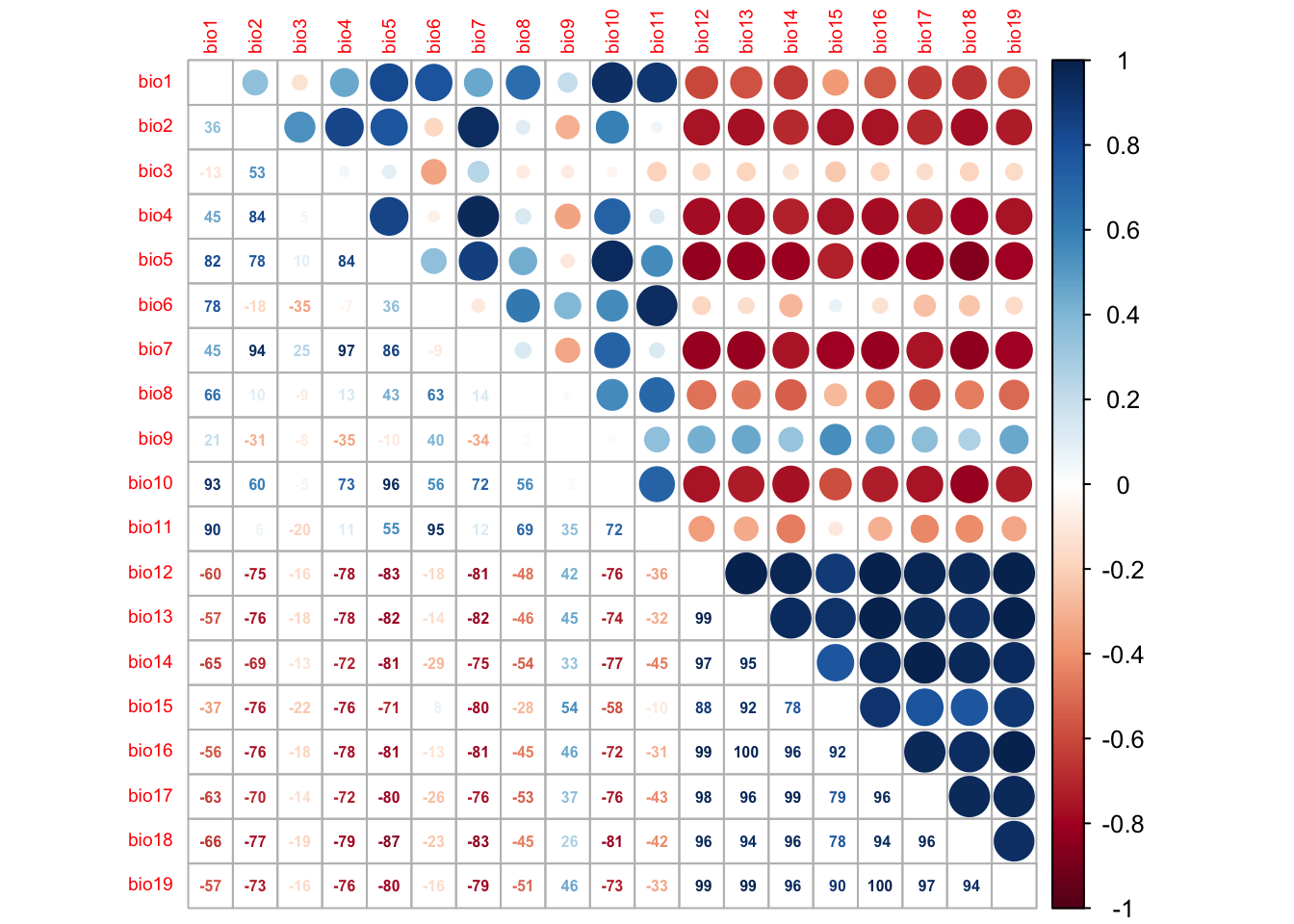RStudio project

Open the RStudio project that we created in the first session. I recommend to use this RStudio project for the entire course and within the RStudio project create separate R scripts for each session.

• Create a new empty R script by going to the tab “File”, select “New File” and then “R script”
• In the new R script, type # Session b3: SDM introduction and save the file in your folder “scripts” within your project folder, e.g. as “b3_SDM_intro.R”

# 1 Introduction

This session will introduce you to simple species distribution models (SDMs). Species distribution models (SDMs) are a popular tool in quantitative ecology (Franklin 2010; Peterson et al. 2011; Guisan, Thuiller, and Zimmermann 2017) and constitute the most widely used modelling framework in global change impact assessments for projecting potential future range shifts of species (IPBES 2016). There are several reasons that make them so popular: they are comparably easy to use because many software packages (e.g. Thuiller et al. 2009; Phillips, Anderson, and Schapire 2006) and guidelines (e.g. Elith, Leathwick, and Hastie 2008; Elith et al. 2011; Merow, Smith, and Silander Jr 2013; Guisan, Thuiller, and Zimmermann 2017) are available, and they have comparably low data requirements.

SDMs relate biodiversity observations (e.g. presence-only, presence/absence, abundance, species richness) at specific sites to the prevailing environmental conditions at those sites. Different statistical and machine-learning algorithms are available for this. Based on the estimated biodiversity-environment relationship, we can make predictions in space and in time by projecting the model onto available environmental layers (Figure 1).Figure 1. Schematic representation of the species distribution modelling concept. First, biodiversity and environmental information are sampled in geographic space. Second, a statistical model (here, generalised linear model) is used to estimate the species-environment relationship. Third, the species–environment relationship can be mapped onto geographic layers of environmental information to delineate the potential distribution of the species. Mapping to the sampling area and period is usually referred to as interpolation, while transferring to a different time period or geographic area is referred to as extrapolation.

We distinguish five main modelling steps for SDMs: (i) conceptualisation, (ii) data preparation, (iii) model fitting, (iv) model assessment, and (v) prediction (Figure 2.). The last step (prediction) is not always part of SDM studies but depends on the model objective (Zurell et al. 2020). Generally, we distinguish three main objectives for SDMs: (a) inference and explanation, (b) mapping and interpolation, and (c) forecast and transfer. I recommend getting more familiar with critical assumptions and modelling decisions by studying the many excellent review articles (Guisan and Zimmermann 2000; Guisan and Thuiller 2005; Elith and Graham 2009) and textbooks on SDMs (Peterson et al. 2011; Franklin 2010; Guisan, Thuiller, and Zimmermann 2017).

I would also like to emphasise that model building is an iterative process and there is much to learn on the way. In consequence, you may want to revisit and improve certain modelling steps, for example improve the spatial sampling design. Because of that I like to regard model building as a cycle rather than a workflow with a pre-defined termination point (Figure 2.)(Zurell et al. 2020).Figure 2. The main modelling cycle in species distribution modelling.

## 1.1 Prac overview

In this session, we will only work with Generalised Linear Models (GLMs) and concentrate on the first three model building steps (Figure 2.)

## 1.2 Generalised linear models (GLMs)

Why do we not simply use linear regression to fit our species-environment relationship? Well, strictly, ordinary least squares (OLS) linear regression is only valid if the response (or rather the error) is normally distributed and ranges ($$-\infty,\infty$$). OLS regression looks like this

$E(Y|X)=\beta X+\epsilon$

where $$E(Y|X)$$ is the conditional mean, meaning the expected value of the response $$Y$$ given the environmental predictors $$X$$ (Hosmer and Lemeshow 2013). $$X$$ is the matrix of predictors (including the intercept), $$\beta$$ are the coefficients for the predictors, and $$\epsilon$$ is the (normally distributed!) error term. $$\beta X$$ is referred to as the linear predictor.

When we want to predict species occurrence based on environment, then the conditional mean $$E(Y|X)$$ is binary and bounded between 0 (absence) and 1 (presence). Thus, the assumptions of OLS regression are not met. GLMs are more flexible regression models that allow the response variable to follow other distributions. Similar to OLS regression, we also fit a linear predictor $$\beta X$$ and then relate this linear predictor to the mean of the response variable using a link function. The link function is used to transform the response to normality. In case of a binary response, we typically use the logit link (or sometimes the probit link). The conditional mean is then given by:

$E(Y|X) = \pi (X) = \frac{e^{\beta X+\epsilon}}{1+e^{\beta X+\epsilon}}$

The logit transformation is defined as: $g(X) = ln \left( \frac{\pi (X)}{1-\pi (X)} \right) = \beta X+\epsilon$

The trick is that the logit, g(X), is now linear in its parameters, is continuous and may range ($$-\infty,\infty$$). GLMs with a logit link are also called logistic regression models.

# 2 Conceptualisation

In the conceptualisation phase, we formulate our main research objectives and decide on the model and study setup based on any previous knowledge on the species and study system. An important point here is whether we can use available data or have to gather own biodiversity (and environmental) data, which would require deciding on an appropriate sampling design. Then, we carefully check the main underlying assumptions of SDMs, for example whether the species is in pseudo-equilibrium with environment and whether the data could be biased in any way (cf. chapter 5 in Guisan, Thuiller, and Zimmermann 2017). The choice of adequate environmental predictors, of modelling algorithms and of desired model complexity should be guided by the research objective and by hypotheses regarding the species-environment relationship. We can divide environmental variables into three types of predictors: resource variables, direct variables and indirect variables (Austin 1980; Guisan and Zimmermann 2000).

## 2.1 Example: Ring Ouzel

We aim at assessing potential climate change effects on the Ring Ouzel (Turdus torquatus) in UK (Figure 3), a typical upland bird. First, we try to make ourselves familiar with Ring Ouzel ecology. For example, the RSPB in UK (The Royal Society for the Protection of Birds) as well as the IUCN (Internatinal Union for the Conservation of Nature) provide some species details on their websites (RSPB, IUCN). Think about which factors could limit the distribution of Ring Ouzel in UK.# 3 Data preparation

In this step, the actual biodiversity and environmental data are gathered and processed. This concerns all data that are required for model fitting but also data that are used for making transfers. In the previous sessions, you have already explored some ways to retrieve species data and environmental data. To simplify matters a little bit, I provide the processed data for this example, which you can download from here (or from the data folder in moodle). The data frame contains ring ouzel presences and absences as well as bioclimatic data.

The ring ouzel occurrence records were obtained from the British breeding and wintering birds citizen science atlas (Gillings et al. 2019). The atlas contains breeding bird records in 20-year cycles (1968-1972, 1988-1991, 2008-2011 and) wintering bird records in 30-year cycles (1981/1982-1983-1984, 2007/2008-2010/2011) at a 10 km spatial resolution throughout Britain, Ireland, the Isle of Man and the Channel Islands. The entire atlas data are available through the British Trust of Ornithology (www.bto.org) and can be downloaded here. I already extracted the confirmed breeding occurrences of the ring ouzel for the most recent atlas period 2008-2011. As absence data I used those locations where no ring ouzel occurrence was reported. Afterwards I spatially thinned the data such that all presence and absence locations are at least 20 km apart. This is important to avoid problems with spatial autocorrelation. The 19 bioclimatic variables were extracted from the worldclim data base (see detail on the variables here).

library(terra)
sp_dat <- read.table('data/Prac3_RingOuzel.txt',header=T)

Inspect the data, and plot the presence and absence locations:

summary(sp_dat)
##     EASTING          NORTHING       Turdus_torquatus       bio1
##  Min.   : 75000   Min.   :  35000   Min.   :0.00000   Min.   : 5.274
##  1st Qu.:265000   1st Qu.: 235000   1st Qu.:0.00000   1st Qu.: 7.784
##  Median :345000   Median : 405000   Median :0.00000   Median : 8.932
##  Mean   :358432   Mean   : 466815   Mean   :0.09901   Mean   : 8.679
##  3rd Qu.:440000   3rd Qu.: 700000   3rd Qu.:0.00000   3rd Qu.: 9.660
##  Max.   :655000   Max.   :1175000   Max.   :1.00000   Max.   :10.841
##       bio2            bio3            bio4            bio5
##  Min.   :3.895   Min.   :29.99   Min.   :290.9   Min.   :14.43
##  1st Qu.:6.404   1st Qu.:36.87   1st Qu.:402.9   1st Qu.:17.53
##  Median :6.972   Median :37.67   Median :430.7   Median :19.01
##  Mean   :6.856   Mean   :37.55   Mean   :427.3   Mean   :19.01
##  3rd Qu.:7.529   3rd Qu.:38.45   3rd Qu.:459.5   3rd Qu.:20.83
##  Max.   :8.453   Max.   :41.14   Max.   :491.7   Max.   :22.91
##       bio6              bio7            bio8             bio9
##  Min.   :-2.8670   Min.   :12.35   Min.   : 1.090   Min.   : 5.019
##  1st Qu.: 0.1564   1st Qu.:17.11   1st Qu.: 4.531   1st Qu.: 6.796
##  Median : 0.8018   Median :18.49   Median : 5.583   Median : 8.898
##  Mean   : 0.7861   Mean   :18.23   Mean   : 5.664   Mean   : 9.150
##  3rd Qu.: 1.3992   3rd Qu.:19.83   3rd Qu.: 6.677   3rd Qu.:10.893
##  Max.   : 4.4728   Max.   :21.40   Max.   :13.413   Max.   :14.624
##      bio10           bio11            bio12            bio13
##  Min.   :10.75   Min.   :0.2874   Min.   : 552.9   Min.   : 51.90
##  1st Qu.:12.99   1st Qu.:3.2112   1st Qu.: 717.6   1st Qu.: 73.81
##  Median :14.33   Median :4.0166   Median : 946.5   Median :105.07
##  Mean   :14.15   Mean   :3.8380   Mean   :1076.8   Mean   :121.29
##  3rd Qu.:15.48   3rd Qu.:4.5036   3rd Qu.:1340.5   3rd Qu.:156.52
##  Max.   :16.90   Max.   :7.0155   Max.   :2749.7   Max.   :330.78
##      bio14            bio15            bio16           bio17
##  Min.   : 33.41   Min.   : 9.668   Min.   :147.3   Min.   :116.0
##  1st Qu.: 44.25   1st Qu.:15.414   1st Qu.:212.7   1st Qu.:146.0
##  Median : 53.75   Median :22.271   Median :300.2   Median :174.1
##  Mean   : 59.95   Mean   :21.785   Mean   :349.2   Mean   :193.9
##  3rd Qu.: 71.71   3rd Qu.:27.119   3rd Qu.:447.5   3rd Qu.:226.7
##  Max.   :127.20   Max.   :36.253   Max.   :966.7   Max.   :407.8
##      bio18           bio19
##  Min.   :140.6   Min.   :128.7
##  1st Qu.:165.8   1st Qu.:188.7
##  Median :203.7   Median :266.7
##  Mean   :223.5   Mean   :310.7
##  3rd Qu.:266.7   3rd Qu.:393.9
##  Max.   :459.3   Max.   :871.1
# Plot GB land mass
plot(bg,col='grey',axes=F,legend=F)

# Plot presences in red and absences in black
plot(extend(terra::rast(sp_dat[,1:3], crs=crs(bg), type='xyz'), bg), col=c('black','red'), legend=F,add=T)# 4 Model fitting

## 4.1 Fitting our first GLM

Before we start into the complexities of the different model fitting steps, let us look at a GLM in more detail. We fit our first GLM with only one predictor assuming a linear relationship between response and predictor. The glm function is contained in the R stats package. We need to specify a formula describing how the response should be related to the predictors, and the data specifying the data frame that contains the response and predictor variables, and a family argument specifying the type of response and the link function. In our case, we use the logit link in the binomial family.

# We first fit a GLM for the bio1 variable assuming a linear relationship:
m1 <- glm(Turdus_torquatus ~ bio1, family="binomial", data= sp_dat)

# We can get a summary of the model:
summary(m1) 
##
## Call:
## glm(formula = Turdus_torquatus ~ bio1, family = "binomial", data = sp_dat)
##
## Deviance Residuals:
##     Min       1Q   Median       3Q      Max
## -1.7195  -0.3760  -0.1635  -0.1003   2.6042
##
## Coefficients:
##             Estimate Std. Error z value Pr(>|z|)
## (Intercept)  10.1088     1.8787   5.381 7.41e-08 ***
## bio1         -1.5579     0.2521  -6.178 6.47e-10 ***
## ---
## Signif. codes:  0 '***' 0.001 '**' 0.01 '*' 0.05 '.' 0.1 ' ' 1
##
## (Dispersion parameter for binomial family taken to be 1)
##
##     Null deviance: 195.68  on 302  degrees of freedom
## Residual deviance: 128.76  on 301  degrees of freedom
## AIC: 132.76
##
## Number of Fisher Scoring iterations: 6

### 4.1.1 Deviance and AIC

Additional to the slope values, there are a few interesting metrics printed in the output called deviance and AIC. These metrics tell us something about how closely the model fits the observed data.

Basically, we want to know whether a predictor is “important”. We thus need to evaluate whether a model including this variable tells us more about the response than a model without that variable (Hosmer and Lemeshow 2013). Importantly, we do not look at the significance level of predictors. Such p-values merely tell us whether the slope coefficient is significantly different from zero. Rather, we assess whether the predicted values are closer to the observed values when the variable is included in the model versus when it is not included.

In logistic regression, we compare the observed to predicted values using the log-likelihood function:

$L( \beta ) = ln[l( \beta)] = \sum_{i=1}^{n} \left( y_i \times ln[\pi (x_i)] + (1-y_i) \times ln[1- \pi (x_i)] \right)$

$$L( \beta )$$ is the Likelihood of the fitted model. From this, we can calculate the deviance $$D$$ defined as:

$D = -2 \times L$

In our model output, the deviance measure is called Residual deviance. This deviance is an important measure used for comparing models. For example, it serves for the calculation of Information criteria that are used for comparing models containing different numbers of parameters. One prominent measure is the Akaike Information Criterion, usually abbreviated as AIC. It is defined as: $AIC = -2 \times L + 2 \times (p+1) = D + 2 \times (p+1)$

where $$p$$ is the number of regression coefficients in the model. AIC thus takes into account model complexity. In general, lower values of AIC are preferable.

We can also use the deviance to calculate the Explained deviance $$D^2$$, which is the amount of variation explained by the model compared to the null expectation: $D^2 = 1 - \frac{D(model)}{D(Null.model)}$ The model output also provides the Null deviance, so we can easily calculate the explained deviance $$D^2$$.

## 4.2 More complex GLMs

We can also fit quadratic or higher polynomial terms (check ?poly) and interactions between predictors:
- the term I()indicates that a variable should be transformed before being used as predictor in the formula
- poly(x,n) creates a polynomial of degree $$n$$: $$x + x^2 + ... + x^n$$
- x1:x2 creates a two-way interaction term between variables x1 and x2, the linear terms of x1 and x2 would have to be specified separately
- x1*x2 creates a two-way interaction term between variables x1 and x2 plus their linear terms
- x1*x2*x3 creates the linear terms of the three variables, all possible two-way interactions between these variables and the three-way interaction

Try out different formulas:

# Fit a quadratic relationship with bio1:
m1_q <- glm(Turdus_torquatus ~ bio1 + I(bio1^2), family="binomial", data= sp_dat)
summary(m1_q)

# Or use the poly() function:
summary( glm(Turdus_torquatus ~ poly(bio1,2) , family="binomial", data= sp_dat) )

# Fit two linear variables:
summary( glm(Turdus_torquatus ~ bio1 + bio8, family="binomial", data= sp_dat) )

# Fit three linear variables:
summary( glm(Turdus_torquatus ~ bio1 + bio8 + bio17, family="binomial", data= sp_dat) )

# Fit three linear variables with up to three-way interactions
summary( glm(Turdus_torquatus ~ bio1 * bio8 * bio17, family="binomial", data= sp_dat) )

# Fit three linear variables with up to two-way interactions
summary( glm(Turdus_torquatus ~ bio1 + bio8 + bio17 +
bio1:bio8 + bio1:bio17 + bio8:bio17,
family="binomial", data= sp_dat) )

## 4.3 Important considerations during model fitting

Model fitting is at the heart of any SDM application. Important aspects to consider during the model fitting step are:

• How to deal with multicollinearity in the environmental data?
• How many variables should be included in the model (without overfitting) and how should we select these?
• Which model settings should be used?
• When multiple model algorithms or candidate models are fitted, how to select the final model or average the models?
• Do we need to test or correct for non-independence in the data (spatial or temporal autocorrelation, nested data)?
• Do we want to threshold the predictions, and which threshold should be used?

More detailed descriptions on these aspects can be found in Franklin (2010) and in Guisan, Thuiller, and Zimmermann (2017).

## 4.4 Collinearity and variable selection

GLMs (and many other statistical models) have problems to fit stable parameters if two or more predictor variables are highly correlated, resulting in so-called multicollinearity issues (Dormann et al. 2013). To avoid these problems here, we start by checking for multi-collinearity and by selecting an initical set of predictor variables. Then, we can fit our GLM including multiple predictors and with differently complex response shapes. This model can then be further simplified by removing “unimportant” predictors.

### 4.4.1 Correlation among predictors

We first check for pairwise correlations among predictors. Generally, correlations below |r|<0.7 are considered unproblematic (or below |r|<0.5 as more conservative threshold).

library(corrplot)

# We first estimate a correlation matrix from the predictors.
# We use Spearman rank correlation coefficient, as we do not know
# whether all variables are normally distributed.
cor_mat <- cor(sp_dat[,-c(1:3)], method='spearman')

# We can visualise this correlation matrix. For better visibility,
# we plot the correlation coefficients as percentages.
corrplot.mixed(cor_mat, tl.pos='lt', tl.cex=0.6, number.cex=0.5, addCoefasPercent=T)Several predictor variables are highly correlated. One way to deal with this issue is to remove the “less important” variable from the highly correlated pairs. For this, we need to assess variable importance.

### 4.4.2 Variable selection: removing highly correlated variables

If no prior knowledge is available on the importance of different predictors, then Dormann et al. (2013) suggest to assess univariate variable importance in terms of AIC (Akaike information criterion) or explained deviance. In practice, this means that we fit a GLM separately for each predictor, assess the importance and then rank the variables according to their univariate importance. Earlier, we already fitted GLMs with linear and quadratic terms and compared AIC. Which one was better?

However, it’s getting a bit more complicated when we want to compare all variables. First, we need to identify all pairs of variables that have correlation |r|>0.7 and remove the less important variable. Dormann et al. (2013) call this the select07 method.

A select07() function is implemented in the package mecofun along with some other useful functions for the entire MGC module. You should already have installed the package from the Univ. Potsdam gitlab during Practical a3.

We load the package and try out the select07() function. Also, check out the help pages for ?select07.

library(mecofun)

# Run select07()
var_sel <- select07(X=sp_dat[,-c(1:3)],
y=sp_dat$Turdus_torquatus, threshold=0.7) # Check out the structure of the resulting object: str(var_sel) ## List of 3 ##$ AIC     : Named num [1:19] 133 136 145 146 150 ...
##   ..- attr(*, "names")= chr [1:19] "bio1" "bio11" "bio10" "bio8" ...
##  $cor_mat : num [1:19, 1:19] 1 0.355 -0.128 0.454 0.821 ... ## ..- attr(*, "dimnames")=List of 2 ## .. ..$ : chr [1:19] "bio1" "bio2" "bio3" "bio4" ...
##   .. ..$: chr [1:19] "bio1" "bio2" "bio3" "bio4" ... ##$ pred_sel: chr [1:6] "bio1" "bio8" "bio14" "bio9" ...
# We extract the names of the weakly correlated predictors ordered by the univariate variable importance in terms of AIC:
pred_sel <- var_sel$pred_sel So, we have now reduced our set of 19 bioclimatic variables to 6 predictors that are only weakly correlated. Now, we need to decide whether all of these should be included in the final model, or if we select only a few, for example the two most important variables in terms of univariate AIC. As a rule of thumb, we should have ten presence points per parameter fitted in the model (Guisan, Thuiller, and Zimmermann 2017). # How many presence points do we have? sum(sp_dat$Turdus_torquatus)
##  30

According to the rule of thumb, we can only include 4 parameters and want to include quadratic terms as well. Thus, for this example, we should only use two predictors. In our case, the best two predictors in terms of univariate AIC were bio1 and bio8.

## 4.5 Model selection

Now that we have selected a set of weakly correlated variables, we can fit the full model and then simplify it. The latter is typically called model selection. Here, I only use the two most important variables and include linear and quadratic terms in the full model.

# Fit the full model:
m_full <- glm( Turdus_torquatus ~ bio1 + I(bio1^2) + bio8 + I(bio8^2),
family='binomial', data=sp_dat)

# Inspect the model:
summary(m_full)
##
## Call:
## glm(formula = Turdus_torquatus ~ bio1 + I(bio1^2) + bio8 + I(bio8^2),
##     family = "binomial", data = sp_dat)
##
## Deviance Residuals:
##      Min        1Q    Median        3Q       Max
## -1.44526  -0.33800  -0.11735  -0.03192   3.01275
##
## Coefficients:
##             Estimate Std. Error z value Pr(>|z|)
## (Intercept)   3.4831    15.6143   0.223    0.823
## bio1         -0.5171     4.6625  -0.111    0.912
## I(bio1^2)    -0.0746     0.2972  -0.251    0.802
## bio8          1.7453     1.5663   1.114    0.265
## I(bio8^2)    -0.2169     0.1676  -1.294    0.196
##
## (Dispersion parameter for binomial family taken to be 1)
##
##     Null deviance: 195.68  on 302  degrees of freedom
## Residual deviance: 122.24  on 298  degrees of freedom
## AIC: 132.24
##
## Number of Fisher Scoring iterations: 8

How much deviance is explained by our model? The function expl_deviance() is also contained in the mecofun package. As explained earlier, we can calculate explained deviance by quantifying how closely the model predictions fit the data in relation to the null model predictions.

# Explained deviance:
expl_deviance(obs = sp_dat$Turdus_torquatus, pred = m_full$fitted)
##  0.3753193

We can simplify the model further by using stepwise variable selection. The function step() uses the AIC to compare different subsets of the model. Specifically, it will iteratively drop variables and add variables until the AIC cannot be improved further (meaning it will not decrease further).

m_step <- step(m_full) 
# Inspect the model:
summary(m_step)
##
## Call:
## glm(formula = Turdus_torquatus ~ I(bio1^2) + bio8 + I(bio8^2),
##     family = "binomial", data = sp_dat)
##
## Deviance Residuals:
##      Min        1Q    Median        3Q       Max
## -1.42982  -0.33815  -0.11284  -0.02967   3.00631
##
## Coefficients:
##             Estimate Std. Error z value Pr(>|z|)
## (Intercept)  1.76080    1.66925   1.055  0.29150
## I(bio1^2)   -0.10745    0.02917  -3.684  0.00023 ***
## bio8         1.61773    1.04632   1.546  0.12208
## I(bio8^2)   -0.20389    0.11734  -1.738  0.08229 .
## ---
## Signif. codes:  0 '***' 0.001 '**' 0.01 '*' 0.05 '.' 0.1 ' ' 1
##
## (Dispersion parameter for binomial family taken to be 1)
##
##     Null deviance: 195.68  on 302  degrees of freedom
## Residual deviance: 122.25  on 299  degrees of freedom
## AIC: 130.25
##
## Number of Fisher Scoring iterations: 8
# Explained deviance:
expl_deviance(obs = sp_dat$Turdus_torquatus, pred = m_step$fitted)
##  0.3752566

The explained deviance of the final model is a tiny bit lower than for the full model, but the final model is more parsimonious.

Exercise:

• Load the second species data set for the Yellowhammer.
• Plot the presences and absences of the Yellowhammer.
• Check for collinearity and reduce the data set to only weakly correlated variables.
• Define a full model with all weakly correlated variables including their linear and quadratic terms.
• Run the full model.
• Simplify the model using stepwise variable selection step()
• Compare the full model and the reduced model in terms of AIC and explained deviance.